Include your answers in this document in the sections below the rubric.

# Rubric

Answer the questions with the data example.

# Height vs Hand Span

In a previous year, this was the procedure for collecting data:

1. Record your height in inches. For example 5’0" is 60 inches.
2. Use a ruler to measure your hand span in centimeters: the distance from the tip of your thumb to pinky finger with your hand splayed as wide as possible.
4. Analysis.
library(tidyverse)
## -- Attaching packages --------------------------------------- tidyverse 1.3.1 --
## v ggplot2 3.3.5     v purrr   0.3.4
## v tibble  3.1.3     v dplyr   1.0.7
## v tidyr   1.1.3     v stringr 1.4.0
## v readr   2.0.0     v forcats 0.5.1
## -- Conflicts ------------------------------------------ tidyverse_conflicts() --
## x dplyr::lag()    masks stats::lag()
library(erikmisc)
## Registered S3 methods overwritten by 'lme4':
##   method                          from
##   cooks.distance.influence.merMod car
##   influence.merMod                car
##   dfbeta.influence.merMod         car
##   dfbetas.influence.merMod        car
## Registered S3 methods overwritten by 'parameters':
##   method                           from
##   as.double.parameters_kurtosis    datawizard
##   as.double.parameters_skewness    datawizard
##   as.double.parameters_smoothness  datawizard
##   as.numeric.parameters_kurtosis   datawizard
##   as.numeric.parameters_skewness   datawizard
##   as.numeric.parameters_smoothness datawizard
##   print.parameters_distribution    datawizard
##   print.parameters_kurtosis        datawizard
##   print.parameters_skewness        datawizard
##   summary.parameters_kurtosis      datawizard
##   summary.parameters_skewness      datawizard
## erikmisc, solving common complex data analysis workflows
##   by Dr. Erik Barry Erhardt <erik@StatAcumen.com>
# Height vs Hand Span
dat_hand <-
na.omit() %>%
mutate(
Gender_M_F = factor(Gender_M_F, levels = c("F", "M"))
)
## Rows: 378 Columns: 6
## -- Column specification --------------------------------------------------------
## Delimiter: ","
## chr (2): Semester, Gender_M_F
## dbl (4): Table, Person, Height_in, HandSpan_cm
##
## i Use spec() to retrieve the full column specification for this data.
## i Specify the column types or set show_col_types = FALSE to quiet this message.
str(dat_hand)
## tibble [237 x 6] (S3: tbl_df/tbl/data.frame)
##  $Semester : chr [1:237] "F15" "F15" "F15" "F15" ... ##$ Table      : num [1:237] 1 1 1 1 1 1 1 1 2 2 ...
##  $Person : num [1:237] 1 2 3 4 5 6 7 8 1 2 ... ##$ Gender_M_F : Factor w/ 2 levels "F","M": 2 1 1 1 2 2 1 2 2 1 ...
##  $Height_in : num [1:237] 69 66 65 62 67 67 65 70 67 63 ... ##$ HandSpan_cm: num [1:237] 21.5 20 20 18 19.8 23 22 21 21.2 16.5 ...
##  - attr(*, "na.action")= 'omit' Named int [1:141] 9 13 14 15 16 17 18 22 23 24 ...
##   ..- attr(*, "names")= chr [1:141] "9" "13" "14" "15" ...

Plot data for Height_in vs HandSpan_cm for Females and Males.

library(ggplot2)
p <- ggplot(dat_hand, aes(x = HandSpan_cm, y = Height_in))
p <- p + theme_bw()
# linear regression fit and confidence bands
p <- p + geom_smooth(method = lm, se = TRUE)
# jitter a little to uncover duplicate points
p <- p + geom_jitter(position = position_jitter(.1), alpha = 0.75)
# separate for Females and Males
p <- p + facet_wrap(~ Gender_M_F, nrow = 1)
print(p)
## geom_smooth() using formula 'y ~ x'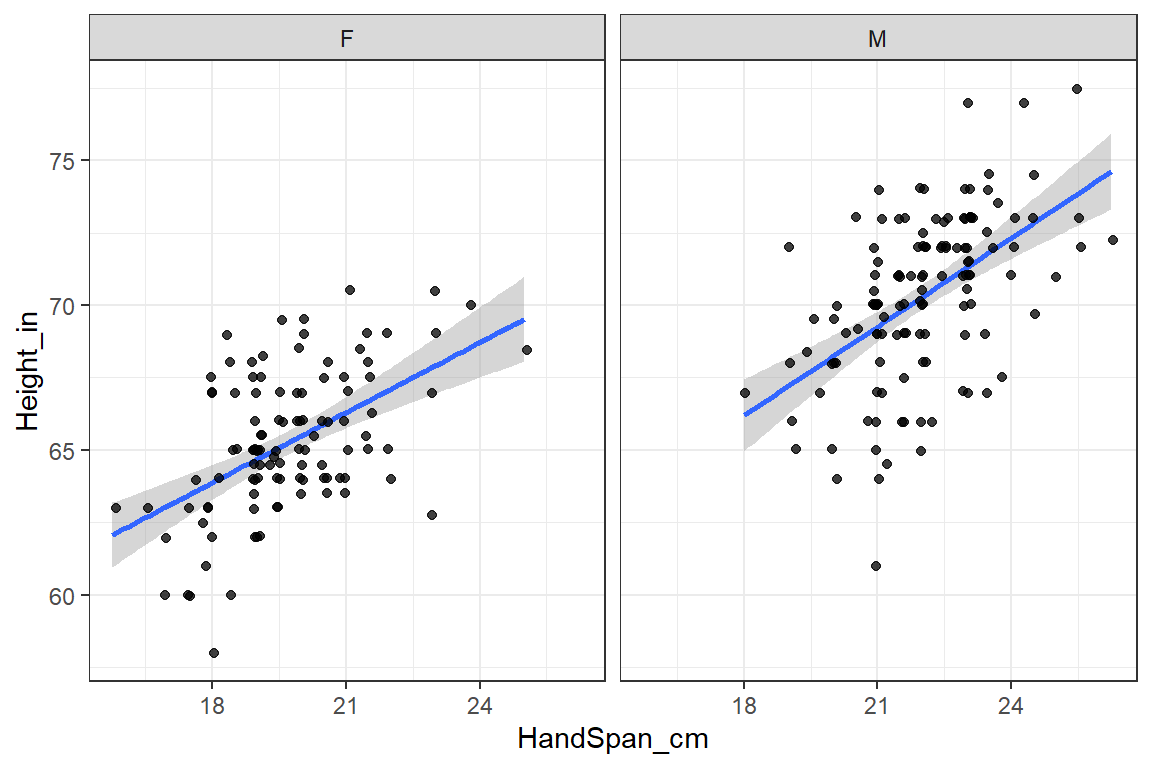Choose either Females or Males for the remaining analysis.

Uncomment one of the Gender_M_F == lines below to choose Females or Males.

# choose one:
dat_use <-
dat_hand %>%
filter(
# Gender_M_F == "F"
# Gender_M_F == "M"
)

Plan:

1. Center the explanatory variable HandSpan_cm,
2. fit a simple linear regression model,
3. check model assumptions,
4. interpret the parameter estimate table, and
5. interpret a confidence and prediction interval.

## Center the explanatory variable HandSpan_cm

Recentering the $$x$$-variable doesn’t change the model, but it does provide an interpretation for the intercept of the model. For example, if you interpret the intercept for the regression lines above, it’s the “expected height for a person with a hand span of zero”, but that’s not meaningful.

Choose a value to center your data on. A good choice is a nice round number near the mean (or center) of your data. This becomes the value for the interpretation of your intercept.

val_center <- 20
dat_use <-
dat_use %>%
mutate(
HandSpan_cm_centered = HandSpan_cm - val_center
)

## Fit a simple linear regression model

# fit model
lm_fit <-
lm(
Height_in ~ HandSpan_cm_centered
, data = dat_use
)

Here’s the data you’re using for the linear regression, with the regression line and confidence and prediction intervals.

library(ggplot2)
p <- ggplot(dat_use, aes(x = HandSpan_cm_centered, y = Height_in))
p <- p + theme_bw()
p <- p + geom_vline(xintercept = 0, alpha = 0.25)
# prediction bands
p <- p + geom_ribbon(aes(ymin = predict(lm_fit, data.frame(HandSpan_cm_centered)
, interval = "prediction", level = 0.95)[, 2],
ymax = predict(lm_fit, data.frame(HandSpan_cm_centered)
, interval = "prediction", level = 0.95)[, 3],)
, alpha=0.1, fill="darkgreen")
# linear regression fit and confidence bands
p <- p + geom_smooth(method = lm, se = TRUE)
# jitter a little to uncover duplicate points
p <- p + geom_jitter(position = position_jitter(.1), alpha = 0.75)
p <- p + labs(
title = "Regression with confidence and prediction bands"
, caption = paste0("Handspan centered at ", val_center, " cm.")
)
print(p)
## geom_smooth() using formula 'y ~ x'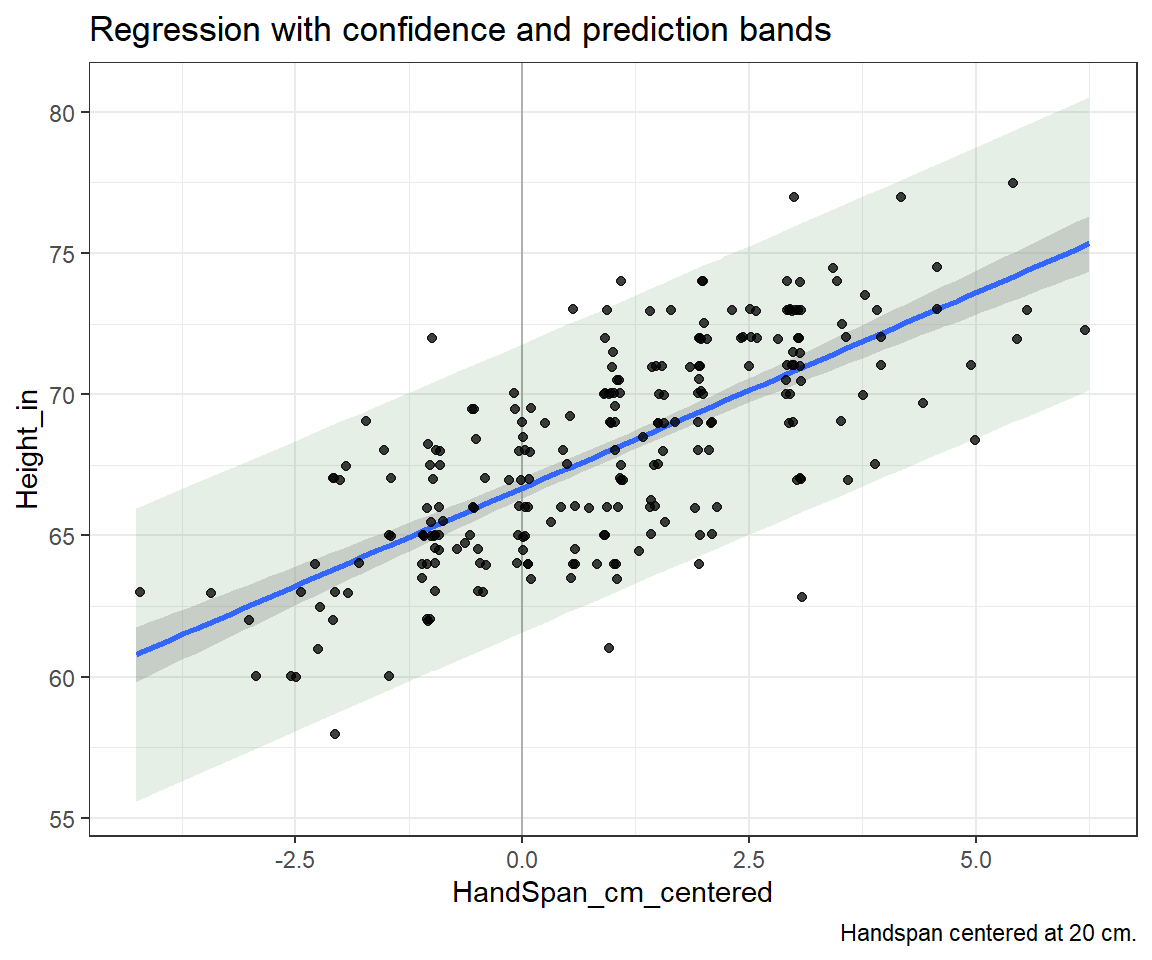## Check model assumptions

Present and interpret the residual plots with respect to model assumptions.

e_plot_lm_diagostics(
lm_fit
#, rc_mfrow    = c(1, 2)
, sw_plot_set = "simple"
)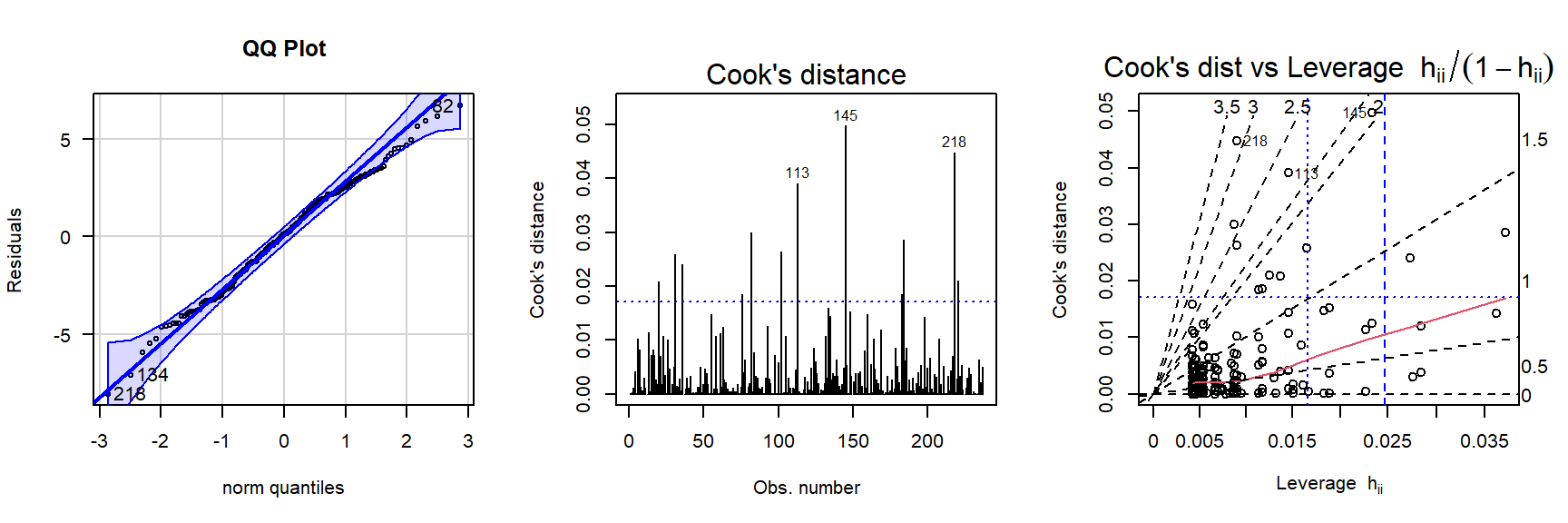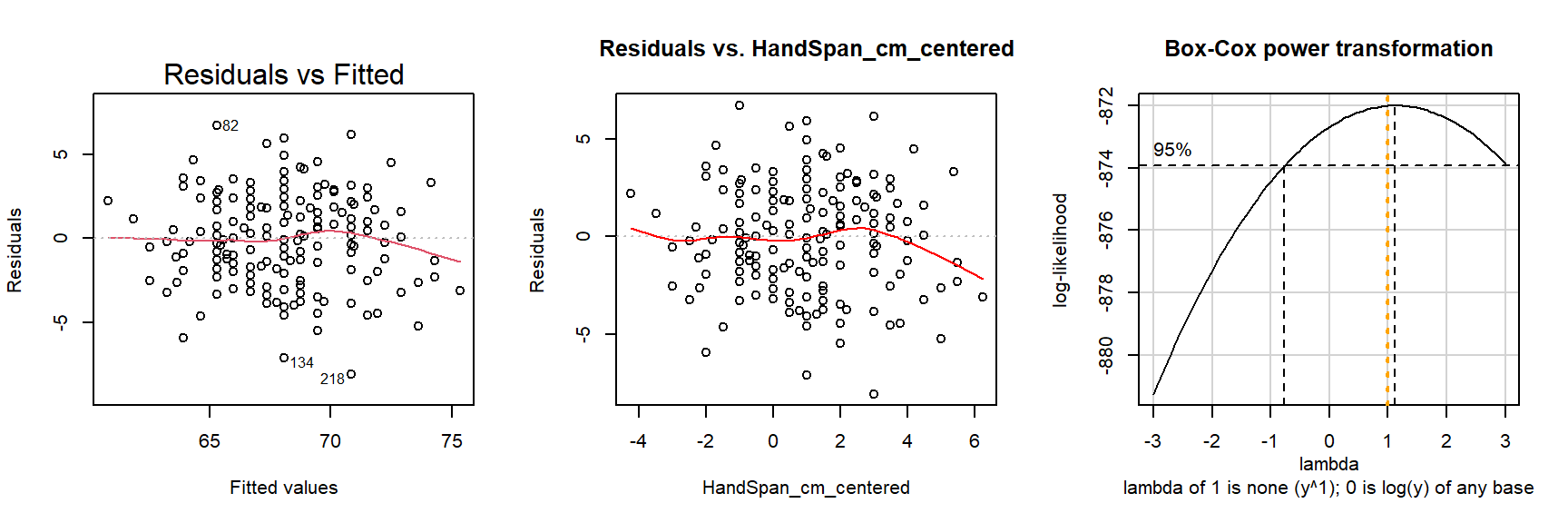(1 p) If the normality assumption seems to be violated, perform a normality test on the standardized residuals.

(1 p) Do the residuals versus the fitted values and HandSpan_cm_centered values appear random? Or is there a pattern?

## Investigate the relative influence of points

Investigate the leverages and Cook’s Distance. There are recommendations for what’s considered large, for example, a $$3p/n$$ cutoff for large leverages, and a cutoff of 1 for large Cook’s D values. I find it more practical to consider the relative leverage or Cook’s D between all the points and worry when there are only a few that are much more influential than others.

Here’s a plot that duplicates a plot above. Here, the observation number is used as both the plotting point and a label.

# plot diagnistics
par(mfrow=c(1,2))

plot(influence(lm_fit)$hat, main="Leverages", type = "n") text(1:nrow(dat_use), influence(lm_fit)$hat, label=paste(1:nrow(dat_use)))
# horizontal line at zero
abline(h = 3 * 2 / nrow(dat_use), col = "gray75")

plot(cooks.distance(lm_fit), main="Cook's Distances", type = "n")
text(1:nrow(dat_use), cooks.distance(lm_fit), label=paste(1:nrow(dat_use)))
# horizontal line at zero
abline(h = qchisq(0.1, 2) / 2, col = "gray75")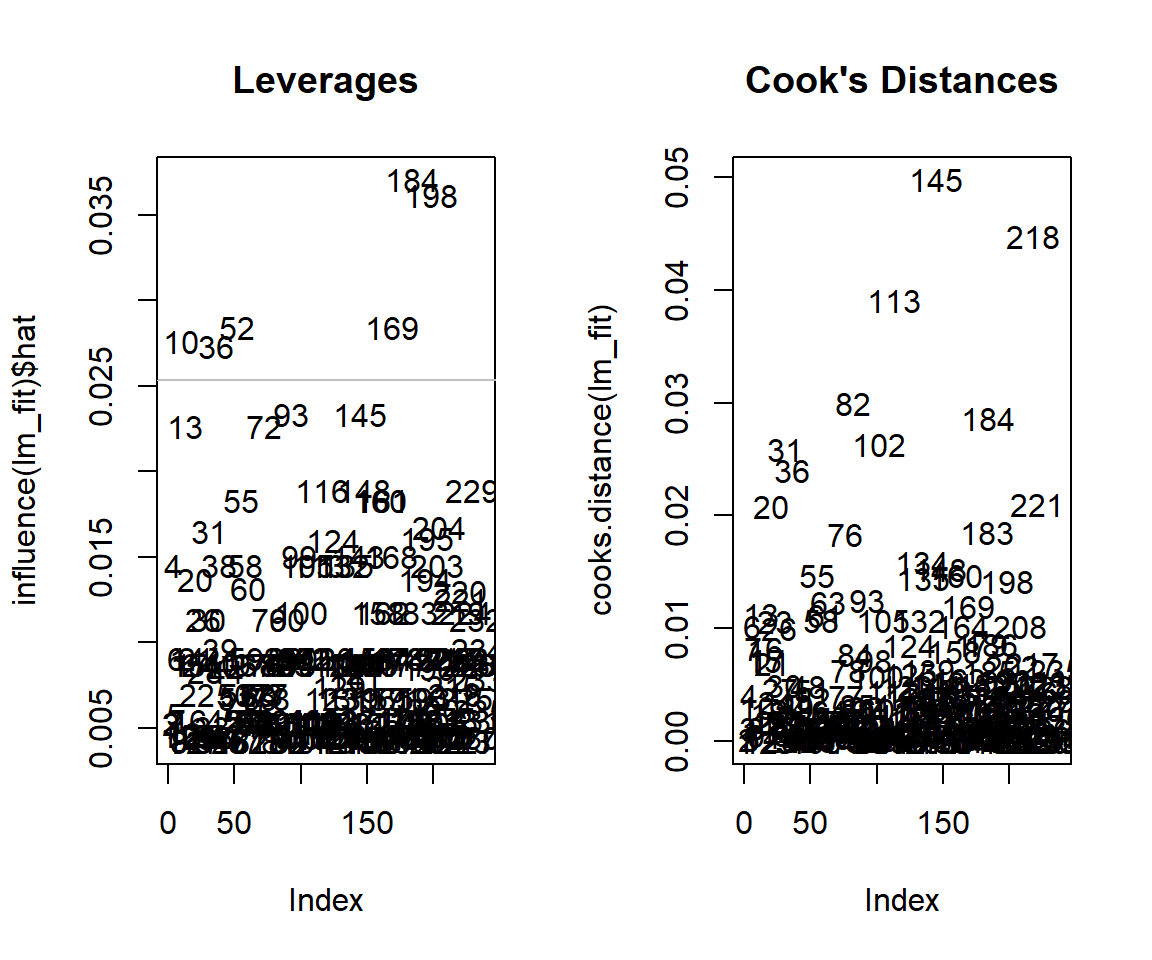(1 p) Interpret the leverages and Cook’s D values with respect to whether any observations are having undue influence on model fit.

## Interpret the parameter estimate table

Here’s the parameter estimate table.

We’re estimating the $$\beta$$ parameter coefficients in the regression model $$y_i = \beta_0 + \beta_1 x_i + e_i$$.

summary(lm_fit)
##
## Call:
## lm(formula = Height_in ~ HandSpan_cm_centered, data = dat_use)
##
## Residuals:
##    Min     1Q Median     3Q    Max
## -8.039 -1.795  0.126  1.933  6.705
##
## Coefficients:
##                      Estimate Std. Error t value Pr(>|t|)
## (Intercept)          66.68105    0.18830  354.13   <2e-16 ***
## HandSpan_cm_centered  1.38596    0.08857   15.65   <2e-16 ***
## ---
## Signif. codes:  0 '***' 0.001 '**' 0.01 '*' 0.05 '.' 0.1 ' ' 1
##
## Residual standard error: 2.585 on 235 degrees of freedom
## Multiple R-squared:  0.5103, Adjusted R-squared:  0.5082
## F-statistic: 244.8 on 1 and 235 DF,  p-value: < 2.2e-16
e_plot_model_contrasts(lm_fit, dat_cont = dat_use)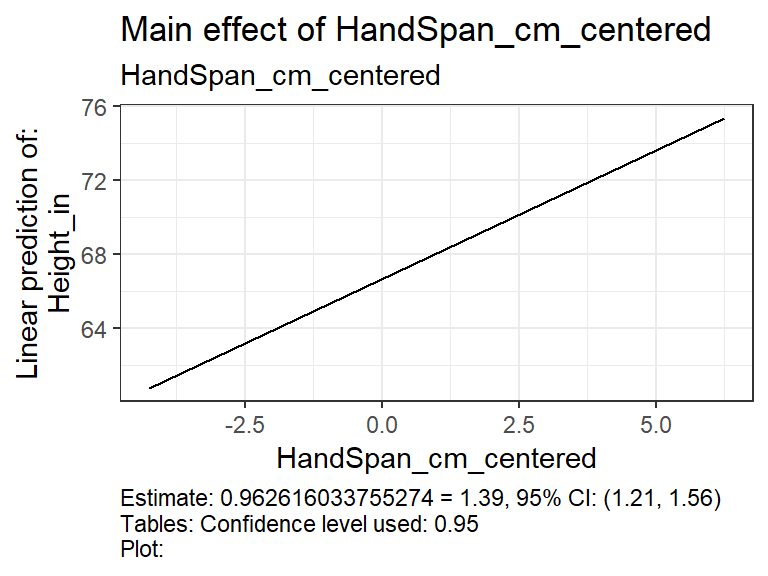(1 p) Assuming the model fits well, complete this equation (fill in the $$\beta$$ values with values from the table) with the appropriate numbers from the table above (3 numbers: each beta and the HandSpan centering value).

The regression line is $$\hat{\textrm{Height_in}} = \hat{\beta}_0 + \hat{\beta}_1 \textrm{(HandSpan_cm - 20)}$$.

(2 p) State the hypothesis test related to the slope of the line, indicate the p-value for the test, and state the conclusion.

Words and notation:

• Words:
• Notation: $$H_0:\beta_? = ?$$ vs $$H_A:\beta_? \ne ?$$

(1 p) Interpret the slope coefficient in the context of the model.

(1 p) State and interpret the $$R^2$$ value.

## Interpret a confidence and prediction interval

Below is a 95% confidence interval (CI) for the mean (the regression line) and a prediction interval (PI) for a new observation at $$\textrm{HandSpan_cm_centered} = -1$$. See how these match up with the plot above.

predict(lm_fit, data.frame(HandSpan_cm_centered = -1)
, interval = "confidence", level = 0.95)
##        fit      lwr      upr
## 1 65.29509 64.81897 65.77121
predict(lm_fit, data.frame(HandSpan_cm_centered = -1)
, interval = "prediction", level = 0.95)
##        fit      lwr      upr
## 1 65.29509 60.18097 70.40921

(1 p) Interpret the CI.

(1 p) Interpret the PI.